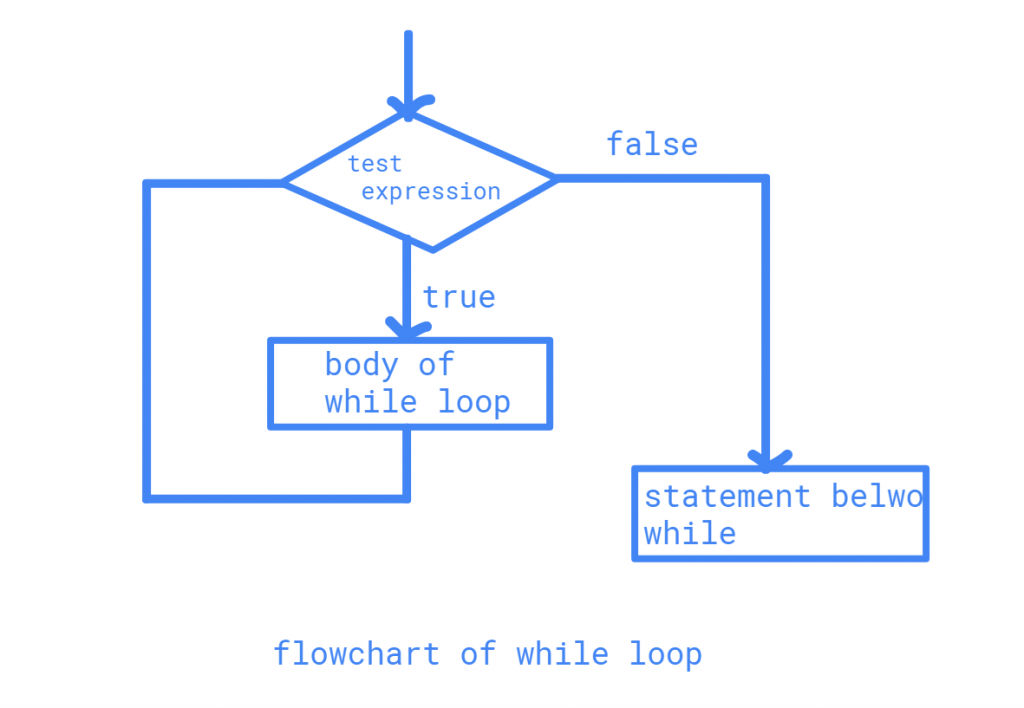# While loop in Javawhile it can be used as an infinite loop too, the while loop executes the statements if the test condition is true. this process continues until the test condition fails(false). let us look into the while loop syntax

syntax

while(test condition)
{
statements(s);
}

here, as long as the condition is satisfied the statements between the braces are executed, let’s look into a program for factorial and flow chart of while loop.we can use increment and decrement statements inside the while loop

flowchartexample

import java.util.*;
class whileloop
{
public static void main(String args[])
{
Scanner s=new Scanner(System.in);
System.out.printf("Enter an integer :");
int n1=s.nextInt();
long factorial=1;
while (n1 > 0)
{
factorial *= n1; // factorial = factorial*number;
--n1;
}
System.out.println(factorial);
s.close();
}
}

output

Enter an integer: 5
Factorial= 120
Last updated on by vishal devxo